# 九、估计图像中的投影关系

• 校准相机
• 计算图像对的基本矩阵
• 使用随机样本共识来匹配图像
• 计算两个图像之间的单应性

# 简介

## 图像形成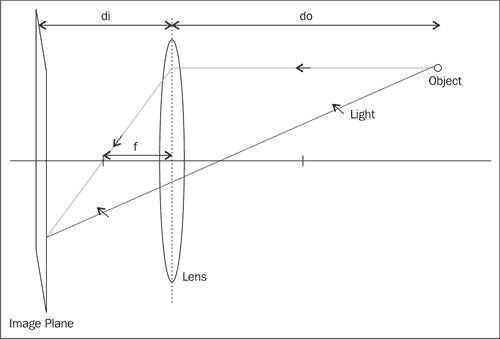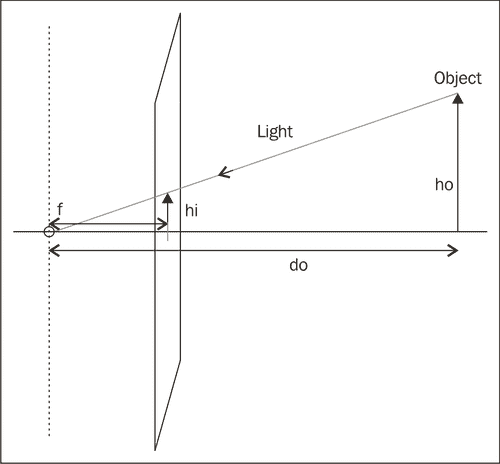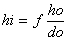# 校准相机

## 操作步骤

OpenCV 建议使用棋盘图案来生成校准所需的 3D 场景点集。 该图案在每个正方形的角上创建点，并且由于该图案是平坦的，因此我们可以自由地假定板位于Z = 0，并且 X 和 Y 轴与网格对齐。 在这种情况下，校准过程仅包括从不同角度向摄像机显示棋盘图案。 这是校准图案图像的一个示例：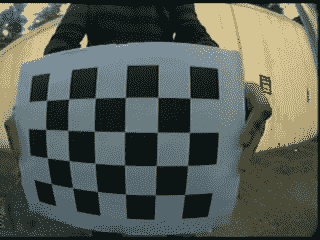// output vectors of image points
std::vector<cv::Point2f> imageCorners;
// number of corners on the chessboard
cv::Size boardSize(6,4);
// Get the chessboard corners
bool found = cv::findChessboardCorners(image,
boardSize, imageCorners);


        //Draw the corners
cv::drawChessboardCorners(image,
boardSize, imageCorners,
found); // corners have been found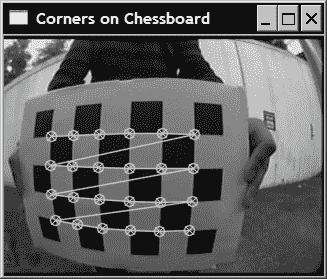class CameraCalibrator {

// input points:
// the points in world coordinates
std::vector<std::vector<cv::Point3f>> objectPoints;
// the point positions in pixels
std::vector<std::vector<cv::Point2f>> imagePoints;
// output Matrices
cv::Mat cameraMatrix;
cv::Mat distCoeffs;
// flag to specify how calibration is done
int flag;
// used in image undistortion
cv::Mat map1,map2;
bool mustInitUndistort;

public:
CameraCalibrator() : flag(0), mustInitUndistort(true) {};


// Open chessboard images and extract corner points
const std::vector<std::string>& filelist,
cv::Size & boardSize) {

// the points on the chessboard
std::vector<cv::Point2f> imageCorners;
std::vector<cv::Point3f> objectCorners;

// 3D Scene Points:
// Initialize the chessboard corners
// in the chessboard reference frame
// The corners are at 3D location (X,Y,Z)= (i,j,0)
for (int i=0; i<boardSize.height; i++) {
for (int j=0; j<boardSize.width; j++) {

objectCorners.push_back(cv::Point3f(i, j, 0.0f));
}
}

// 2D Image points:
cv::Mat image; // to contain chessboard image
int successes = 0;
// for all viewpoints
for (int i=0; i<filelist.size(); i++) {

// Open the image

// Get the chessboard corners
bool found = cv::findChessboardCorners(
image, boardSize, imageCorners);

// Get subpixel accuracy on the corners
cv::cornerSubPix(image, imageCorners,
cv::Size(5,5),
cv::Size(-1,-1),
cv::TermCriteria(cv::TermCriteria::MAX_ITER +
cv::TermCriteria::EPS,
30,      // max number of iterations
0.1));  // min accuracy

//If we have a good board, add it to our data
if (imageCorners.size() == boardSize.area()) {

// Add image and scene points from one view
successes++;
}

}

return successes;
}


// Add scene points and corresponding image points
void CameraCalibrator::addPoints(const std::vector<cv::Point2f>& imageCorners, const std::vector<cv::Point3f>& objectCorners) {

// 2D image points from one view
imagePoints.push_back(imageCorners);
// corresponding 3D scene points
objectPoints.push_back(objectCorners);
}


// Calibrate the camera
// returns the re-projection error
double CameraCalibrator::calibrate(cv::Size &imageSize)
{
// undistorter must be reinitialized
mustInitUndistort= true;

//Output rotations and translations
std::vector<cv::Mat> rvecs, tvecs;

// start calibration
return
calibrateCamera(objectPoints, // the 3D points
imagePoints,  // the image points
imageSize,    // image size
cameraMatrix, // output camera matrix
distCoeffs,   // output distortion matrix
rvecs, tvecs, // Rs, Ts
flag);        // set options
}


// remove distortion in an image (after calibration)
cv::Mat CameraCalibrator::remap(const cv::Mat &image) {

cv::Mat undistorted;

if (mustInitUndistort) { // called once per calibration

cv::initUndistortRectifyMap(
cameraMatrix,  // computed camera matrix
distCoeffs,    // computed distortion matrix
cv::Mat(),     // optional rectification (none)
cv::Mat(),     // camera matrix to generate undistorted
image.size(),  // size of undistorted
CV_32FC1,      // type of output map
map1, map2);   // the x and y mapping functions

mustInitUndistort= false;
}

// Apply mapping functions
cv::remap(image, undistorted, map1, map2,
cv::INTER_LINEAR); // interpolation type

return undistorted;
}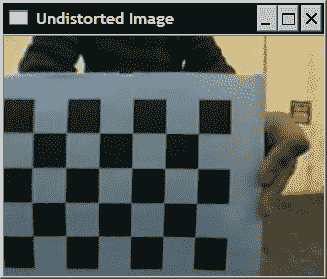## 工作原理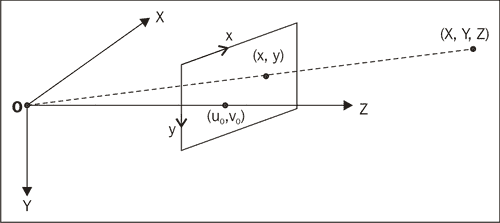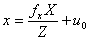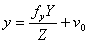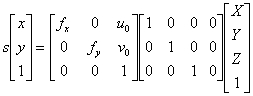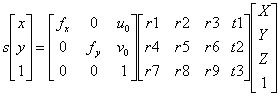# 计算图像对的基本矩阵

## 准备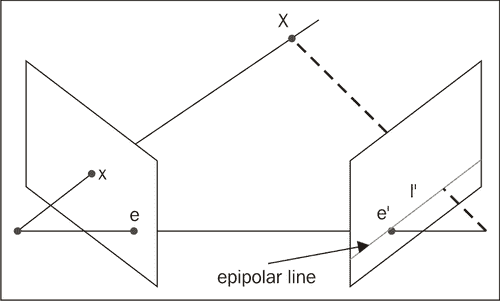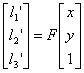## 操作步骤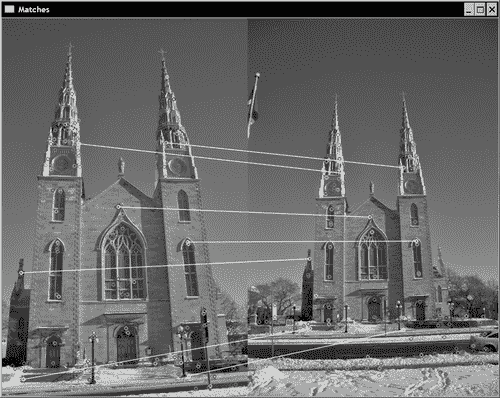// Convert keypoints into Point2f
std::vector<cv::Point2f> selPoints1, selPoints2;
cv::KeyPoint::convert(keypoints1,selPoints1,pointIndexes1);
cv::KeyPoint::convert(keypoints2,selPoints2,pointIndexes2);


   // Compute F matrix from 7 matches
cv::Mat fundemental= cv::findFundamentalMat(
cv::Mat(selPoints1), // points in first image
cv::Mat(selPoints2), // points in second image
CV_FM_7POINT);       // 7-point method


   // draw the left points corresponding epipolar
// lines in right image
std::vector<cv::Vec3f> lines1;
cv::computeCorrespondEpilines(
cv::Mat(selPoints1), // image points
1,                   // in image 1 (can also be 2)
fundemental, // F matrix
lines1);     // vector of epipolar lines

// for all epipolar lines
for (vector<cv::Vec3f>::const_iterator it= lines1.begin();
it!=lines1.end(); ++it) {

// draw the line between first and last column
cv::line(image2,
cv::Point(0,-(*it)/(*it)),
cv::Point(image2.cols,-((*it)+
(*it)*image2.cols)/(*it)),
cv::Scalar(255,255,255));
}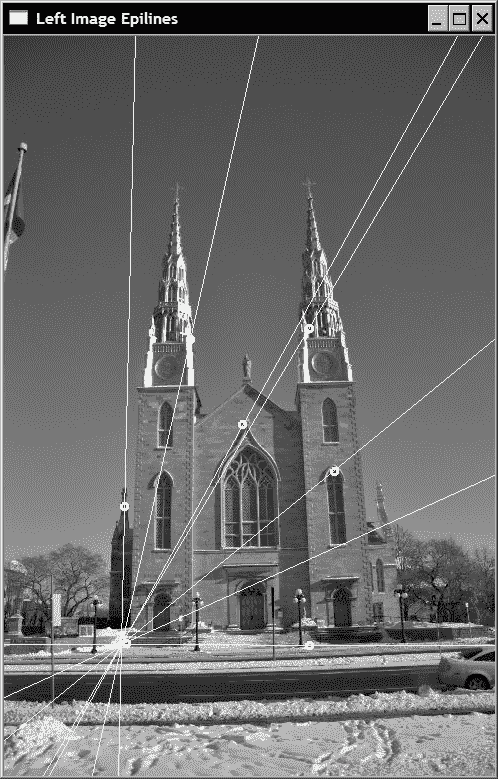## 工作原理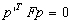## 另见

The book by R. Hartley and A. Zisserman, Multiple View Geometry in Computer Vision, Cambridge University Press, 2004 is the most complete reference on projective geometry in computer vision.


# 使用随机样本共识匹配图像

## 操作步骤

class RobustMatcher {

private:

// pointer to the feature point detector object
cv::Ptr<cv::FeatureDetector> detector;
// pointer to the feature descriptor extractor object
cv::Ptr<cv::DescriptorExtractor> extractor;
float ratio; // max ratio between 1st and 2nd NN
bool refineF; // if true will refine the F matrix
double distance; // min distance to epipolar
double confidence; // confidence level (probability)

public:

RobustMatcher() : ratio(0.65f), refineF(true),
confidence(0.99), distance(3.0) {

// SURF is the default feature
detector= new cv::SurfFeatureDetector();
extractor= new cv::SurfDescriptorExtractor();
}


     // Set the feature detector
void setFeatureDetector(
cv::Ptr<cv::FeatureDetector>& detect) {

detector= detect;
}

// Set the descriptor extractor
void setDescriptorExtractor(
cv::Ptr<cv::DescriptorExtractor>& desc) {

extractor= desc;
}


     // Match feature points using symmetry test and RANSAC
// returns fundemental matrix
cv::Mat match(cv::Mat& image1,
cv::Mat& image2, // input images
// output matches and keypoints
std::vector<cv::DMatch>& matches,
std::vector<cv::KeyPoint>& keypoints1,
std::vector<cv::KeyPoint>& keypoints2) {

// 1a. Detection of the SURF features
detector->detect(image1,keypoints1);
detector->detect(image2,keypoints2);

// 1b. Extraction of the SURF descriptors
cv::Mat descriptors1, descriptors2;
extractor->compute(image1,keypoints1,descriptors1);
extractor->compute(image2,keypoints2,descriptors2);

// 2\. Match the two image descriptors

// Construction of the matcher
cv::BruteForceMatcher<cv::L2<float>> matcher;

// from image 1 to image 2
// based on k nearest neighbours (with k=2)
std::vector<std::vector<cv::DMatch>> matches1;
matcher.knnMatch(descriptors1,descriptors2,
matches1, // vector of matches (up to 2 per entry)
2);        // return 2 nearest neighbours

// from image 2 to image 1
// based on k nearest neighbours (with k=2)
std::vector<std::vector<cv::DMatch>> matches2;
matcher.knnMatch(descriptors2,descriptors1,
matches2, // vector of matches (up to 2 per entry)
2);        // return 2 nearest neighbours

// 3\. Remove matches for which NN ratio is
// > than threshold

// clean image 1 -> image 2 matches
int removed= ratioTest(matches1);
// clean image 2 -> image 1 matches
removed= ratioTest(matches2);

// 4\. Remove non-symmetrical matches
std::vector<cv::DMatch> symMatches;
symmetryTest(matches1,matches2,symMatches);

// 5\. Validate matches using RANSAC
cv::Mat fundemental= ransacTest(symMatches,
keypoints1, keypoints2, matches);

// return the found fundemental matrix
return fundemental;
}


     // Clear matches for which NN ratio is > than threshold
// return the number of removed points
// (corresponding entries being cleared,
// i.e. size will be 0)
int ratioTest(std::vector<std::vector<cv::DMatch>>
&matches) {

int removed=0;

// for all matches
for (std::vector<std::vector<cv::DMatch>>::iterator
matchIterator= matches.begin();
matchIterator!= matches.end(); ++matchIterator) {

// if 2 NN has been identified
if (matchIterator->size() > 1) {

// check distance ratio
if ((*matchIterator).distance/
(*matchIterator).distance > ratio) {

matchIterator->clear(); // remove match
removed++;
}

} else { // does not have 2 neighbours

matchIterator->clear(); // remove match
removed++;
}
}

return removed;
}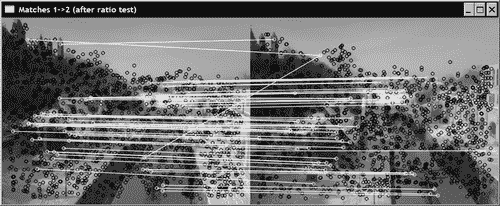// Insert symmetrical matches in symMatches vector
void symmetryTest(
const std::vector<std::vector<cv::DMatch>>& matches1,
const std::vector<std::vector<cv::DMatch>>& matches2,
std::vector<cv::DMatch>& symMatches) {

// for all matches image 1 -> image 2
for (std::vector<std::vector<cv::DMatch>>::
const_iterator matchIterator1= matches1.begin();
matchIterator1!= matches1.end(); ++matchIterator1) {

// ignore deleted matches
if (matchIterator1->size() < 2)
continue;

// for all matches image 2 -> image 1
for (std::vector<std::vector<cv::DMatch>>::
const_iterator matchIterator2= matches2.begin();
matchIterator2!= matches2.end();
++matchIterator2) {

// ignore deleted matches
if (matchIterator2->size() < 2)
continue;

// Match symmetry test
if ((*matchIterator1).queryIdx ==
(*matchIterator2).trainIdx  &&
(*matchIterator2).queryIdx ==
(*matchIterator1).trainIdx) {

symMatches.push_back(
cv::DMatch((*matchIterator1).queryIdx,
(*matchIterator1).trainIdx,
(*matchIterator1).distance));
break; // next match in image 1 -> image 2
}
}
}
}


     // Identify good matches using RANSAC
// Return fundemental matrix
cv::Mat ransacTest(
const std::vector<cv::DMatch>& matches,
const std::vector<cv::KeyPoint>& keypoints1,
const std::vector<cv::KeyPoint>& keypoints2,
std::vector<cv::DMatch>& outMatches) {

// Convert keypoints into Point2f
std::vector<cv::Point2f> points1, points2;
for (std::vector<cv::DMatch>::
const_iterator it= matches.begin();
it!= matches.end(); ++it) {

// Get the position of left keypoints
float x= keypoints1[it->queryIdx].pt.x;
float y= keypoints1[it->queryIdx].pt.y;
points1.push_back(cv::Point2f(x,y));
// Get the position of right keypoints
x= keypoints2[it->trainIdx].pt.x;
y= keypoints2[it->trainIdx].pt.y;
points2.push_back(cv::Point2f(x,y));
}

// Compute F matrix using RANSAC
std::vector<uchar> inliers(points1.size(),0);
cv::Mat fundemental= cv::findFundamentalMat(
cv::Mat(points1),cv::Mat(points2), // matching points
inliers,      // match status (inlier or outlier)
CV_FM_RANSAC, // RANSAC method
distance,     // distance to epipolar line
confidence);  // confidence probability

// extract the surviving (inliers) matches
std::vector<uchar>::const_iterator
itIn= inliers.begin();
std::vector<cv::DMatch>::const_iterator
itM= matches.begin();
// for all matches
for ( ;itIn!= inliers.end(); ++itIn, ++itM) {

if (*itIn) { // it is a valid match

outMatches.push_back(*itM);
}
}

if (refineF) {
// The F matrix will be recomputed with
// all accepted matches

// Convert keypoints into Point2f
// for final F computation
points1.clear();
points2.clear();

for (std::vector<cv::DMatch>::
const_iterator it= outMatches.begin();
it!= outMatches.end(); ++it) {

// Get the position of left keypoints

float x= keypoints1[it->queryIdx].pt.x;
float y= keypoints1[it->queryIdx].pt.y;
points1.push_back(cv::Point2f(x,y));
// Get the position of right keypoints
x= keypoints2[it->trainIdx].pt.x;
y= keypoints2[it->trainIdx].pt.y;
points2.push_back(cv::Point2f(x,y));
}

// Compute 8-point F from all accepted matches
fundemental= cv::findFundamentalMat(
cv::Mat(points1),cv::Mat(points2), // matches
CV_FM_8POINT); // 8-point method
}

return fundemental;
}


   // Prepare the matcher
RobustMatcher rmatcher;
rmatcher.setConfidenceLevel(0.98);
rmatcher.setMinDistanceToEpipolar(1.0);
rmatcher.setRatio(0.65f);
cv::Ptr<cv::FeatureDetector> pfd=
new cv::SurfFeatureDetector(10);
rmatcher.setFeatureDetector(pfd);

// Match the two images
std::vector<cv::DMatch> matches;
std::vector<cv::KeyPoint> keypoints1, keypoints2;
cv::Mat fundemental= rmatcher.match(image1,image2,
matches, keypoints1, keypoints2);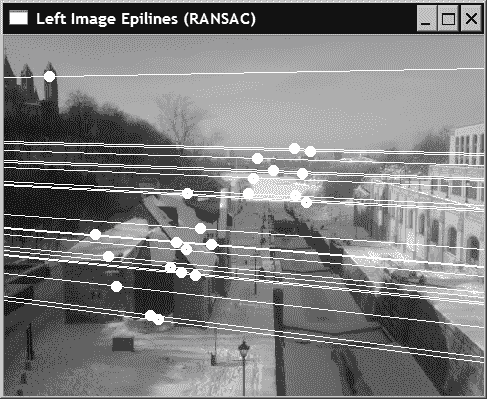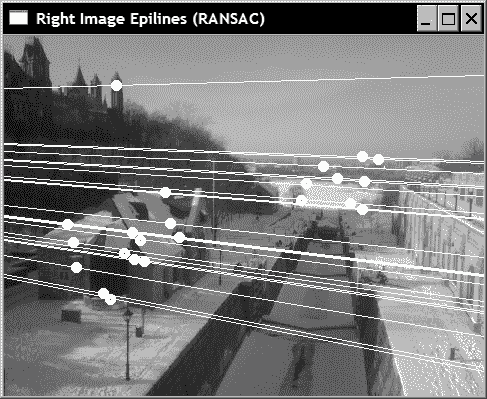## 工作原理

RANSAC 算法旨在从可能包含多个异常值的数据集中估计给定的数学实体。 想法是从集合中随机选择一些数据点，然后仅使用这些数据点进行估计。 选择的点数应该是估计数学实体所需的最小点数。 在基本矩阵的情况下，该最小数目为 8 个匹配对（实际上，可以是 7 个匹配，但是 8 点线性算法的计算速度更快）。 一旦从这些随机的 8 个匹配项中估计出了基本矩阵，就将对照该集合中得出的对极约束，测试匹配集中所有其他匹配项。 识别出满足此约束的所有匹配项（即，对应特征与其对极线相距不远的匹配项）。 这些匹配形成计算出的基本矩阵的支持集

RANSAC 算法背后的中心思想是，支持集越大，计算出的矩阵就是正确矩阵的可能性就越高。 显然，如果一个（或多个）随机选择的匹配项是错误的匹配项，则计算出的基本矩阵也将是错误的，并且其支持集会很小。 重复此过程多次，最后，将保留最大支持的矩阵为最可能的矩阵。

# 计算两个图像之间的单应性

## 准备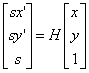## 操作步骤

   // Find the homography between image 1 and image 2
std::vector<uchar> inliers(points1.size(),0);
cv::Mat homography= cv::findHomography(
cv::Mat(points1), // corresponding
cv::Mat(points2), // points
inliers,      // outputted inliers matches
CV_RANSAC,   // RANSAC method
1.);         // max distance to reprojection point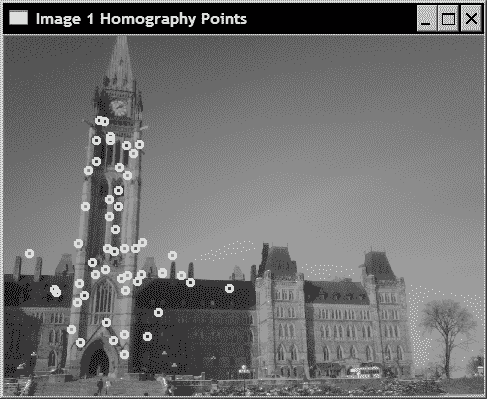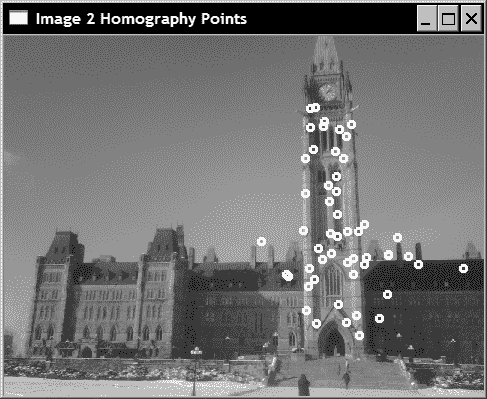// Draw the inlier points
std::vector<cv::Point2f>::const_iterator itPts=
points1.begin();
std::vector<uchar>::const_iterator itIn= inliers.begin();
while (itPts!=points1.end()) {

// draw a circle at each inlier location
if (*itIn)
cv::circle(image1,*itPts,3,
cv::Scalar(255,255,255),2);
++itPts;
++itIn;
}

itPts= points2.begin();
itIn= inliers.begin();
while (itPts!=points2.end()) {

// draw a circle at each inlier location
if (*itIn)
cv::circle(image2,*itPts,3,
cv::Scalar(255,255,255),2);
++itPts;
++itIn;
}


   // Warp image 1 to image 2
cv::Mat result;
cv::warpPerspective(image1, // input image
result,         // output image
homography,      // homography
cv::Size(2*image1.cols,
image1.rows)); // size of output image


   // Copy image 1 on the first half of full image
cv::Mat half(result,cv::Rect(0,0,image2.cols,image2.rows));
image2.copyTo(half); // copy image2 to image1 roi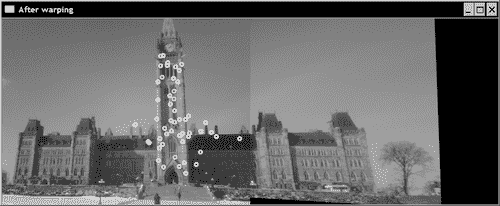## 工作原理

cv::findHomography计算的单应性是将第一图像中的点映射到第二图像中的点的单应性。 实际上，为了将图像 1 的点转移到图像 2 所需要的是逆单应性。 这正是函数cv::warpPerspective在默认情况下所做的，也就是说，它使用提供的单应性的倒数作为输入来获取输出图像每个点的颜色值。 当输出像素转移到输入图像外部的点时，黑色值（0）会简单地分配给该像素。 请注意，如果要在像素传输过程中使用直接单应性而不是反向单应性，则可以将可选标志cv::WARP_INVERSE_MAP指定为cv::warpPerspective中的可选第五个参数。